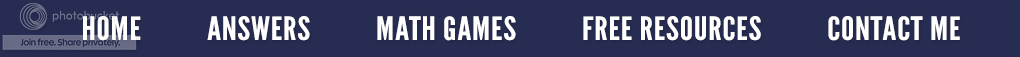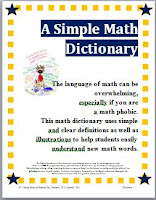### Helping Students to Think Mathematically

What does it mean to think mathematically?

It means using math vocabulary, language and symbols to describe or interpret mathematical concepts, procedures and to discover relationships among ideas.  Therefore when a student problem solves, they use previous knowledge, skills, and understanding of concepts to solve a problem.  This process might include formulating problems, applying a variety of strategies, or interpreting results.

What can we do to help our students become better mathematical thinkers?

We can teach and model problem solving strategies.  We can remember and plan our lessons to involve the three stages of conceptual development: concrete, pictorial, and abstract. We can have the students talk or write about how they got an answer either with the class or with a partner.  We can use writing in the mathematics classroom (such as math journals) to allow the students to practice expository writing and show their understanding.  We can exhibit math word walls and have the students use the glossary in their book to write and define terms.

We can also create a positive and safe classroom atmosphere for problem solving...

By being enthusiastic and allowing the students to take risks without consequences.  By emphasizing the process as well as the answer, the students may be willing to try unconventional or different ways to solve the problem.  I always tell my students that there isn't just one right way to get an answer which surprises many of them.  In fact, this is one of the posters that hangs in my classroom.

As math teachers, let's continue to emphasize problem solving so that all students will acquire confidence in using mathematics meaningfully. But most of all, let's have fun while we are doing it!
﻿\$8.00 on TPT
If you are interested in having a math dictionary for your students, check out A Simple Math Dictionary. It is a 30 page student dictionary that uses easy and clear definitions as well as formulas and examples so that students can learn and understand new math words without difficulty or cumbersome language. Most definitions include diagrams and/or illustrations for the visual learner. Over 300 common math terms are organized alphabetically for quick reference.